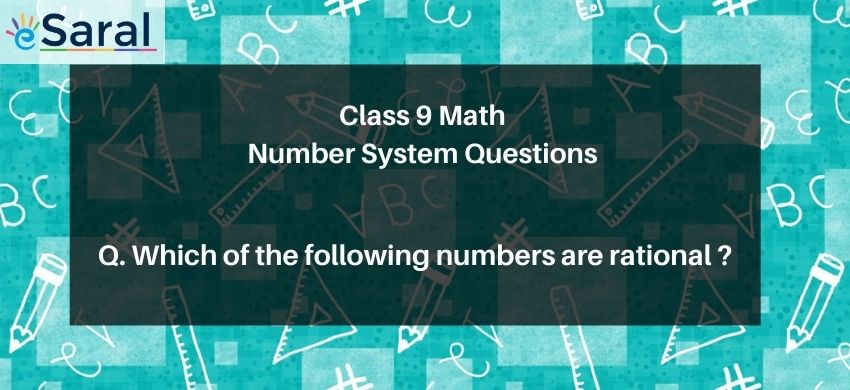Download the eSaral app and start learning from Kota's top IITians and doctors.

# Which of the following numbers are rational ?# Question : Which of the following numbers are rational ?

1. $1$
2. $-6$
3. $3 \frac{1}{2}$
4. All above are rational
Solution :
The correct option is 4. All above are rational
None of the number is irrational as every number can be expressed in the form of $\frac{\mathrm{p}}{\mathrm{q}}$, where $\mathrm{q} \neq 0$.